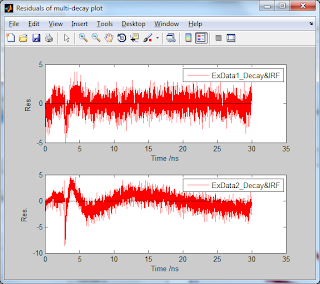### Fit fluorescence intensity decays

#### Time-resolved emission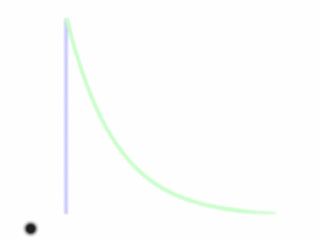Time-resolved fluorescence measurements can be used to determine the excited state lifetime(s) of a fluorophore. In time-resolved fluorescence the sample is repeatedly excited using short light pulses (blue) and the subsequent emission of photons (green) is detected at a photodetector positioned perpendicularly to the excitation beam.

#### Deconvolution of fluorescence decay profiles

In practice, the excitation pulse is not a delta-function and the instrumentation additionally has a certain electronic response time. This is quantified by the instrument response function, IRF or L(t), which is the response profile of the instrument to a purely scattering solution. If L(t) is considered to be a series of delta-excitation pulses with varying amplitude, then the measured intensity at time t, N(t), is the sum of responses to each delta-excitation pulse up until t. Thus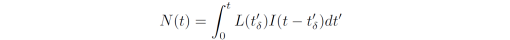where I(t-t') denotes the fluorescence intensity from the sample at time t, originating as a response to a delta-excitation pulse at time t' and with amplitude L(t). I(t) is described physically using the fluorescence intensity decay models

The equation above is called the convolution integral and the task is to determine the model, I(t), which yields the best overall fit between N(t) and L(t). In DecayFit, and most other software, this fitting procedure is achieved using iterative reconvolution with a least squares analysis. In least-squares the "goodness-of-fit" parameter chi-square is minimized by iteratively optimizing the parameter values of the intensity decay model. There is no actual deconvolution taking place. Rather, the IRF is convoluted with the simulated decay before evaluating chi-square. Chi-square, in turn, contains the squared difference between all measured data points and the modeled decay, each of which is weighted according to the expected standard deviation of the measured intensity and (for the reduced chi-square) the total number of data points: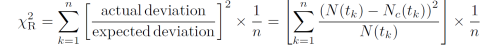Here, N(tk) is the measured counts at time tk, Nc(tk) is the modeled number of counts at time tk, and n is the number of data points.

A good fit is characterized by a value of chi-square close to 1 and the weighted residual between the measured and calculated decay curve should be randomly distributed around 0.

#### Fit time-resolved intensity decays using DecayFit

DecayFit can fit multiple data sets in one click.

1. Select the files you wish to analyse and their associated instrument response function in the data listboxes (note you can only specify one IRF at a time which will be used for all the selected decay data sets). See information on accepted input data formats here.

All of the selected decays and the IRF are plotted in the graph window. Note that if only one decay is selected the parameter table contains both start guesses as well as lower and upper parameter boundaries. If multiple decays are selected the parameter table contains the start guesses of the individual decays.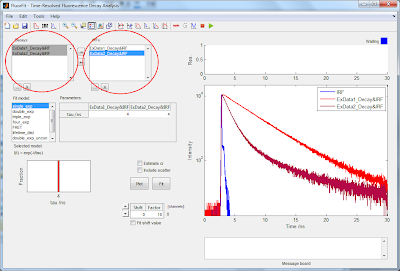2. Select the model to fit from the 'Fit model' listbox. In the MATLAB version of DecayFit the fit model functions are located in the 'library/decaymodels' folder and can be modified at will. The mathematical expression of the model is displayed below the model listbox.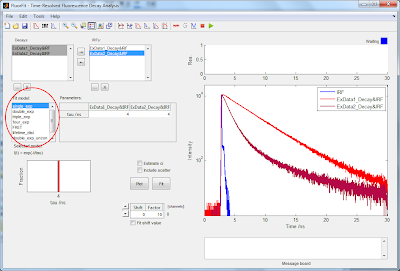3. Specify the initial parameter value guesses and their lower and upper bounds in the parameter table. You can press the Plot button to plot a decay with the specified parameters.

Note, again, that if multiple decays are selected the data table lists the start guesses of the individual decays. If you need to specify upper and lower parameter boundaries for each decay, select the decays from the decays listbox one at a time.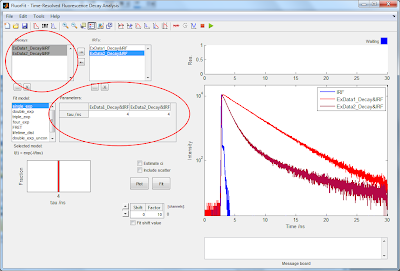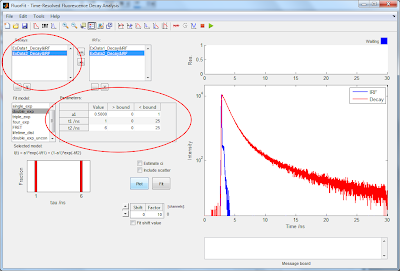5. Specify whether to include the channel shift in between the IRF and the decays as a fitting parameter. The shift value will be optimized within the specified interval at a resolution of 1/Factor channels, where the Factor is specified in the Shift panel. A shift of 1 with a Factor of 10 thus corresponds to a shift of 1/10 channel.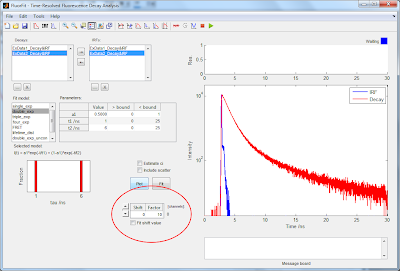6. Choose whether to include scatter in the fitting. In DecayFit scatter is modeled by adding a fraction of the IRF to the convoluted decay.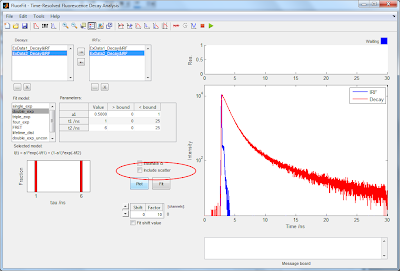7. Choose whether to estimate the 95% confidence intervals (CI) of the fitted parameters using the Jacobian matrix of the fit. Estimating the CI from the Jacobian is not as accurate as calculating the full Chi-square surface. The confidence intervals will be displayed in the message board.8. To run the fit press the Fit button or the green triangle in the Toolbar.9. The fitted parameters are updated in the parameter table and the fits will be plotted in the graph window. The fitted lifetimes and their relative fraction in the decay is visualized in the bar plot to the left.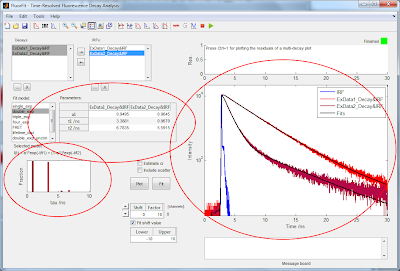Again, if multiple data sets are selected from the Decays listbox only the optimized parameters of the first of the selected sets are being displayed in the table. In order to get all the information of the individual fits select the data sets one by one in the Decays listbox. The parameter table is updated according to the selection, the chi-square of the fit is shown in the top of the graph window, and the residuals are plotted above the graph.
If multiple data sets are selected the individual residuals can be plotted by pressing Ctrl+1.